# Initialize Anomaly Detection Model (One-Class SVM) VI

LabVIEW 2018 Analytics and Machine Learning Toolkit Help

Edition Date: July 2018

Part Number: 377059B-01

»View Product InfoDownload Help (Windows Only)

Owning Palette: Anomaly Detection VIs

Requires: Analytics and Machine Learning Toolkit

Initializes the hyperparameters of the one-class support vector machine (SVM) algorithm. This VI uses the nu-SVM algorithm.

Use the one-class SVM model to estimate the boundary of a high-dimensional distribution. The one-class SVM algorithm trains models on data that has only one class.

Example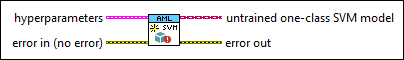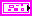hyperparameters specifies the hyperparameters of the one-class SVM model.nu specifies the nu parameter for the one-class SVM model. The nu parameter is both a lower bound for the number of samples that are support vectors and an upper bound for the number of samples that are on the wrong side of the hyperplane. The default is 0.1. The nu parameter must be in the range [0,1].

For example, if nu is 0.05, the training samples that are wrongly classified are not allowed to take up more than 5 percent of all training samples. Also, at least 5 percent of the training samples are support vectors.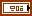kernel settings specifies settings to configure the kernel function.

This VI supports the following kernel functions:

Kernel functionDefinitionDescription
Linear function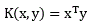x and y are the input sample vectors.
Polynomial function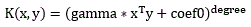x and y are the input sample vectors; gamma, coef0, and degree are the algorithm coefficients.
Radial basis function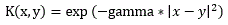x and y are the input sample vectors.
Sigmoid functionx and y are the input sample vectors; gamma and coef0 are the algorithm coefficients.type specifies the type of the kernel function.

 0 Linear (default)—Linear function 1 Polynomial—Polynomial function 2 RBF—Radial basis function 3 Sigmoid—Sigmoid function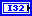degree specifies the degree coefficient of the kernel function. The default is 3.gamma specifies the gamma coefficient of the kernel function. The default is 1.coef0 specifies the coef0 coefficient of the kernel function. The default is 0.tolerance specifies the tolerance for the stopping criteria. The default is 0.001.max iteration specifies the maximum number of optimization iterations for the stopping criteria. The default is 100000. The model fitting stops if the number of optimization iterations reaches max iteration.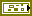error in describes error conditions that occur before this node runs. This input provides standard error in functionality.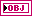untrained one-class SVM model returns the initialized one-class SVM model for training.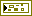error out contains error information. This output provides standard error out functionality.

## Example

Refer to the Anomaly Detection (Training) VI in the labview\examples\AML\Anomaly Detection directory for an example of using the Initialize Anomaly Detection Model (One-Class SVM) VI.